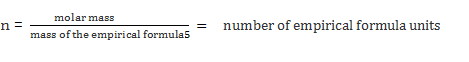# Molecular Formula

## Molecular formula

The molecular formula is the expression of the number of atoms of each element in one molecule of a compound.

The molecular formula definition is the formula showing the actual number of each atom in a molecule.

When the molar mass value is known, the Molecular Formula is calculated by the empirical formula.

####The molecular formula is often same as empirical formula or an exact multiple of it.

Example 1

Caffeine has the following composition: 49.48% of carbon, 5.19% of hydrogen, 16.48% of oxygen and 28.85% of nitrogen. The molecular weight is 194.19 g/mol. Find out the molecular and empirical formula.

Solution

Step 1

Multiply percent composition with the molecular weight

Carbon – 194.19 x 0.4948 = 96.0852

Hydrogen – 194.19 x 0.0519 = 10.07846

Oxygen –  194.19 x 0.1648 = 32.0025

Nitrogen – 194.19 x 0.2885 = 56.0238

Step 2

Divide each value by the atomic weight

Carbon : 96.0852 / 12.011 = 7.9997

Hydrogen : 10.07846 / 1.008 = 9.998

Oxygen : 32.0025 / 15.9994 = 2.000

Nitrogen : 56.0238 / 14.0067 = 3.9997

Step 3

Round off the values to closest whole number

8: Carbon

10: Hydrogen

2: Oxygen

4: Nitrogen

Hence, the molecular formula is C8H10N4O2.

Step 4

Since 2 is the common factor among 8, 10, 4 and 2.

The empirical formula is C4H5N2O.

Example 2

A compound contains 86.88% of carbon and 13.12% of hydrogen, and the molecular weight is 345. What will be the empirical and molecular formula of the compound?

Solution

Step 1

Carbon : 345 x 0.8688 = 299.736

Hydrogen : 345 x 0.1312 = 45.264

Step 2

Carbon : 299.736 / 12.011 = 24.995

Hydrogen : 45.264 / 1.008 = 44.91

Step 3

Molecular formula is C25H45

Step 4

Since 5 is the common factor, the empirical formula is C5H9

To know more examples and practice questions on molecular formulas, please visit Byju’s.com